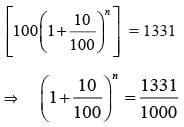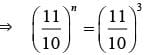## 20 Questions MCQ Test Mathematics Olympiad Class 7 | Olympiad Test: Compound Interest

Description
Attempt Olympiad Test: Compound Interest | 20 questions in 40 minutes | Mock test for Class 7 preparation | Free important questions MCQ to study Mathematics Olympiad Class 7 for Class 7 Exam | Download free PDF with solutions
QUESTION: 1

### Find compound interest on Rs 7500 at 4% per annum for 2 years, compounded annually.

Solution:

Amount  = Rs [7500 × (1 + 4/100)²]
= Rs (7500 × 26/25 × 26/25)
= Rs 8112
C.I. = Rs (8112 – 7500)
= Rs 612

QUESTION: 2

### Find the compound interest on Rs 16,000 at 20% per annum for 9 months, compounded quarterly.

Solution:

Principal = Rs 16, 000;
Time = 9 months = 3 quarters;
Amount = Rs [16000 × (1+5/100)³]
= [16000 × 21/20 × 21/20 × 21/20]
= Rs 18522
C.I = Rs (18522 – 16000)
= Rs 2522

QUESTION: 3

### Simple interest on a certain sum of money for 3 years at 8% per annum is half the compound interest on Rs 4000 for 2 years at 10% per annum. The sum placed on simple interest is:

Solution:

C.I. = Rs [4000 × (1+10/100)² – 4000]
= Rs (4000 × 11/10 × 11/10 – 4000)
= Rs 840
Sum = Rs [420 × 100 /3 × 8] = Rs 1750

QUESTION: 4

Anil invested an amount of Rs 8000 in a fixed deposit scheme for 2 years at compound interest rate 5% per annum. How much amount will Anil get on maturity of the fixed deposit?

Solution:

Amount  = Rs [8000 × (1+5/100)²]
= Rs [8000 × 21/20 × 21/20]
= Rs 8820

QUESTION: 5

The present worth of Rs 169 due in 2 years at 4% per annum compound interest is:

Solution:

Present worth = Rs [169/(1+4/100)²]
= Rs (169 × 25/26 × 25/26)
= Rs 156.25

QUESTION: 6

On a sum of money, the simple interest for 2 years is Rs 660, while the compound interest is Rs 696.30, the rate of interest being the same in both the cases. The rate of interest is:

Solution:

Difference in C.I and S.I for 2 years
= Rs (6 96.30 – 660)
= Rs 36.30
S.I for one year =Rs 330
S.I on Rs 330 for 1 year = Rs 36.30
Rate = (100 × 36.30/330 × 1)% = 11%

QUESTION: 7

The difference between simple interest and compound interest on Rs 1200 for one year at 10% per annum reckoned half yearly is

Solution:

S.I = Rs  (1200 × 10 × 1/100)
= Rs 1 20
C.I = Rs  [1200 × (1+5/100)² – 1200]
= Rs 123
Difference = Rs [123 – 120] = Rs 3

QUESTION: 8

A sum of money invested at compound interest amounts to Rs 800 in 3 years and to Rs 840 in 4 years. The rate of interest per annum is

Solution:

S.I. on Rs 800 for 1 year = Rs [840 – 800]
= Rs 40
Rate = (100 × 40/800 × 1)%
= 5%

QUESTION: 9

If the simple interest on a sum of money for 2 years at 5% per annum is Rs 50, what is the compound interest on the same at the same rate and for the same time?

Solution:

Sum = Rs (50 × 100/2×5)
= Rs 500
Amount = [Rs (500 × (1 + 5/100)²]
= Rs (500 × 21/20 × 21/20)
= Rs 551.25
C.I = Rs (551.25 – 500)
= Rs 51.25

QUESTION: 10

In what time will Rs 1000 become Rs 1331 at 10% per annum compounded annually?

Solution:

Principal = Rs 1000
Amount = Rs 1331
Rate = Rs 10% p.a.
Let the time be n years. ThenTherefore n =  3 years.

QUESTION: 11

If the simple interest on a sum of money at 5% per annum for 3 years is Rs 1200, find the compound interest on the same sum for the same period at the same rate.

Solution:

Clearly, Rate = 5% p.a.
Time = 3 years
S.I = Rs 1200.
So, Principal = Rs (100 × 1200/3× 5)
= Rs 8000
Amount = Rs [8000 × (1+ 5/100)³]
= Rs (8000 × 21/20 × 21/20 × 21/20)
= Rs 9261
C.I = Rs (9261 – 8000)
= Rs 1261

QUESTION: 12

What will be the compound interest on a sum of Rs 25,000 after 3 years at the rate of 12% per annum?

Solution:

Amount = Rs (25000 × (1+12/100)³
= Rs (25000 × 28/25 × 28/25 × 28/25)
= Rs 35123.20.
C.I = Rs (35123.20 – 25000)
= Rs 10123.20

QUESTION: 13

The compound interest on Rs 30,000 at 7% per annum is Rs 4347. The period (in years) is

Solution:

Amount = Rs (30000 + 4347)
= Rs 34347
Let the time be n years.
Then, 30000(1+7/100)n = 34347
⇒ (1+7/100)n  = 34347/3000
⇒ (107/100)n = 11449/1000
⇒(107/100)n = (107/100)2
Therefore  = 2 years

QUESTION: 14

The difference between compound interest and simple interest on an amount of Rs 15,000 for 2 years is Rs 96. What is the rate of interest per annum?

Solution:

[15000 (1+ r/100)2 – 15000] – (15000 × r × 2)/100 = 96
By solving the above equation
r2 = (96 × 2)/3  = 64
Therefore r =  8%

QUESTION: 15

The compound interest on a certain sum for 2 years at 10% per annum is Rs 525. The simple interest on the same sum for double the time at half the rate percent per annum is:

Solution:

Let the sum be Rs P.
Then, [P (1 + 10/100)2 – P] = 525
P = (11/10)2 – 1 = 525
P = (525 × 100)/21 = 2500
Therefore, sum = Rs 2500
S.I. = (2500 × 5 × 4)/100 = Rs 500

QUESTION: 16

At what rate of compound interest per annum will a sum of Rs 1200 become Rs 1348.32 in 2 years?

Solution:

Let the rate be r% p.a.
Then, 1200 × (1 + R/100)2 =1348.32
(1 + R/100)2 = 11236/10000
(1 + R/100)2 = (106/100)2
Therefore r = 6%

QUESTION: 17

The least number of complete years in which a sum of money invested at 20% compound interest will be more than double is:

Solution:

P(1 + 20/100)n > 2P (6/5)n > 2
Now, (6/5 × 6/5 × 6/5 × 6/5) > 2
So, n = 4 years

QUESTION: 18

The effective annual rate of interest corresponding to a nominal rate of 6% per annum payable half-yearly is:

Solution:

Amount of Rs 100 for 1 year when compounded half-yearly
= Rs [100 × (1 + 3/100)2]
= Rs 106.09
Effective rate = (106.09 – 100)% = 6.09%

QUESTION: 19

There is 60% increase in an amount in 6 years at simple interest. What will be the compound interest of Rs 12,000 after 3 years at the same rate?

Solution:

Let P = Rs 100. Then, S.I. Rs 60 and
T = 6 years.
∴ R = 100 × 60 / 100 × 6 = 10% p.a.
Now, P = Rs 12000 T = 3 years and
R = 10% p.a.
∴ C.I.  = Rs [12000 × (1 +10/100)3 – 1]
= 3972

QUESTION: 20

A bank offers 5% compound interest calculated on half-yearly basis. A customer deposits Rs 1600 each on 1st January and 1st July of a year. At the end of the year, the amount he would have gained by way of interest is:

Solution:

Amount = [Rs1600 × (1 + 5/2 ×100)2 +Rs 1600 × (1 +5/2 ×100)]
= Rs 3321
∴ C.I.= Rs (3321 – 3200) = Rs 121Use Code STAYHOME200 and get INR 200 additional OFF Use Coupon Code